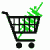February 7, 2023

# Convert Watts to Amps

## How to convert Watts to Amps or Amps to Watts or Volts to Watts

Basics

You cannot convert watts to amps, since watts are power and amps are coulombs per second (like converting gallons to miles). HOWEVER, if you have at least least two of the following three: amps, volts or watts then the missing one can be calculated. Since watts are amps multiplied by volts, there is a simple relationship between them.

However, In some engineering disciplines the volts are more or less fixed, for example in house wiring, automotive wiring, or telephone wiring. In these limited fields technicians often have charts that relate amps to watts and this has caused some confusion. What these charts should be titled is "conversion of amps to watts at a fixed voltage of 110 volts" or "conversion of watts to amps at 13.8 volts," etc.

 Some tidbits of information that you might need a refresher on:To convert mA to A (milliamps to amps) 1000mA = 1Ato convert µA to A (microamps to amps) 1000,000 µA = 1ATo converter µA to mA (microamps to milliamps) 1000µA = 1mA To convert mW to W (milliwatts to watts) 1000mW = 1WTo converter µW to W (microwatts to watts) 1,000,000 µW = 1W

The Following Equations can be used to convert between amps, volts, and watts.
Converting Watts to Amps

The conversion of Watts to Amps at fixed voltage is governed by the equation Amps = Watts/Volts

For example 12 watts/12 volts = 1 amp
Converting Amps to Watts

The conversion of Amps to Watts at fixed voltage is governed by the equation Watts = Amps x Volts

For example 1 amp * 110 volts = 110 watts

Converting Watts to Volts

The conversion of Watts to Volts at fixed amperage is governed by the equation Volts = Watts/Amps

For example 100 watts/10 amps = 10 volts

Converting Volts to Watts

The conversion of Volts to Watts at fixed amperage is governed by the equation Watts = Amps x Volts

For example 1.5 amps * 12 volts = 18 watts

Converting Volts to Amps at fixed wattage

The conversion of Volts to Amps if the wattage is known is governed by the equations Amps = Watts/Volts

For example 120 watts/110 volts = 1.09 amps

Converting Amps to Volts at fixed wattage

The conversion of Amps to Volts if the wattage is know is governed by the equation Volts = Watts/Amps

For Example, 48 watts / 12 Amps = 4 Volts

Converting Volts to Amps at a fixed resistance

If you know the volts and the load of the resistance the amps are found by Ohm's law: Amps = Volts / Resistance

Converting Amps to Volts at fixed resistance

If you know the amps and the resistance Ohm's law becomes Volts = Amps * Resistance

## Explanation

Amps are how many electrons flow past a certain point per second. It is equal to one coulomb of charge per second, or 6.24 x 10^18 electrons per second. Volts is a measure of how much force that each electron is under, which we call "potential". Power (watts) is volts times amps. A few electrons under a lot of potential can supply a lot of power, or a lot of electrons at a low potential can supply the same power. Think of water in a hose. A gallon a minute (think amps) just dribbles out if it is under low pressure (think low voltage). But if you restrict the end of the hose, letting the pressure build up, the water can have more power (like watts), even though it is still only one gallon a minute. In fact the power can grow enormous as the pressure builds, to the point that a water knife can cut a sheet of glass. In the same manner as the voltage is increased a small amount of current can turn into a lot of watts.

This is also why increasing the volts does not necessarily increase the available power. Power is amps times volts, so if you double the volts you halve the amps unless something in your circuit actually creates power, such as a battery, solar panel or nuclear power plant.

Convert amps to watts. Convert watts to amps. Convert watts to volts, convert volts to watts, convert amps to volts at fixed wattage. How to convert watt to amps. How do I convert amps to watt? Amps converting watt. Volt to watt conversion.Thank you for considering PowerStream

 PowerStream Technology 1163 South 1680 West Orem, Utah 84058 USA Phone: 801-764-9060 Fax: 801-764-9061 © Copyright 2000, 2002, 2003, 2004, 2005, 2006, 2008,2009, 2010, 2011, 2012, 2013, 2014, 2015, 2016, 2017, 2018, 2019, 2020, 2021, 2022 Lund Instrument Engineering, Inc. All rights reserved. Google Author Verification